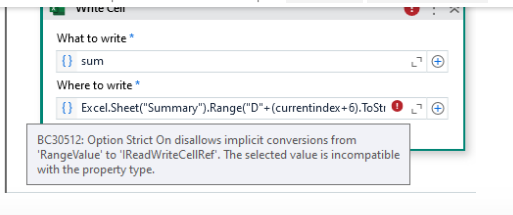# How to get the value in the particular cell dynamically

How to get the cell value after it because sum in a particular
how to get the sum value in every sheet

@anjani_priya

Cheers

Yon can try this

In row(0).ToString… put your Column Name

currentSum = dtCurrentSheet.AsEnumerable().Where(Function(row) Not row(0).ToString().IsNullOrEmpty()).Sum(Function(row) Convert.ToDouble(row(0)))

there is a column called total stock
the column value should be sum and enter at the main sheet
like that it has 21 sheets
every sheet of total stock should be sum and add in main sheet linely

this is the sheet which contains data
the F column should sum and enter in the summary sheet but already the value is there in D column how to sum and enter the value in summary sheet

@anjani_priya

To get sum of a column in datatable you can use the below formula in assign the output would be of double type so create sum as double…then paste it or write it wherever you need

`Sum = dt.AsEnumerable.Sum(function(x) CDBL(x("ToTal Stock").ToString))`

Cheers

Hi @anjani_priya ,
You can use write cell with formunal Sum
regards,

there are 21 sheets how to sum for 21 sheets and pass the values linely in summary this

i have tried this but how to pass the values dynamically

@anjani_priya

Loop through the sheets use the above formula inside loop

And then to write use currentindex to identify the row

In weite cell use `Excel.Sheet("SheetName").Range("D" + (currentindex+6).ToString)`

This will change the cell to d6,d7 and so on as the sheets change in loop

Cheers

@anjani_priya

You are using classic write cell use modern

Cheers

@anjani_priya

Where to write is where you need to write the excel prt what to write is the sum.ToString

Cheers

You are using Write Cell activity so give this

Excel.Sheet(“Summary”).Cell(“D”+(currentindex+6).ToString… like thisIn place of Range use Cell

@anjani_priya